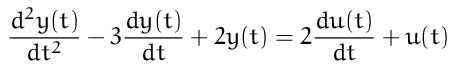# Typesetting differential equations

Posted on September 18, 2017

This semester I am teaching a course involving linear differential equations, so I have typeset many differential equations that look like this:Believe me, writing such equations by hand gets tedious very quickly. So, I wanted a macro `\LDE` (for Linear Differential Equation), so that I could just type

``````\LDE{1, -3, 2}{2, 1}
``````

and get the above result. This post describes how to write such a macro using LuaTeX + ConTeXt.

I’ll explain the implementation using a top-down approach. Writing macros in TeX is tough, so let’s delegate all the high lifting to Lua. So, we define a ConTeXt command that simply invokes a Lua function with the appropriate arguments. This can be done using:

``````\startluacode
-- ... lua function LDE to be added ...
interfaces.definecommand {
name      = "LDE",
macro     = LDE,
arguments = {
{"content", "list" },
{"content", "list" },
}
}
\stopluacode
``````

The `name` argument specifies the name of the TeX command (`\LDE` in this case); the `macro` argument specifies the name of the Lua function (`LDE` in this case) which gets called when the TeX macro is invoked; the `arguments` tables specifies the list of arguments of the TeX macros. We specify that the macro has two arguments, both of them are `content` arguemnts (that is, the macro is invoked as `\LDE{...}{...}`) and each arguement should be passed on to the Lua function as a `list` (i.e., a Lua table of strings; other options include `hash` (for key-value list), `number`, and `string`).

Now all we need to do is define the function `LDE`, which takes two comma separated lists as arguments and typesets the resulting differential equation. The basic logic is straight forward. Suppose we have defined a function `derivative` that typesets a derivative (taking care of adding a `+` sign if the coefficient of an intermediate term is positive; not showing the coefficient if the coefficient is `+1` or `-1`; and not showing the term if the coefficient is `0`). Then, the `LDE` function is:

``````\startluacode
-- Lua function derivative to be added

local LDE = function(LHS, RHS)
for i = 1, #LHS do
context(derivative(LHS[i], "y(t)", #LHS - i, i == 1))
end

context("=")
for i = 1, #RHS do
context(derivative(RHS[i], "u(t)", #RHS - i, i == 1))
end
end

interfaces.definecommand { ... }
\stopluacode
``````

The last term in the call to the `derivative` function passes a flag that indicates whether or not the term is the first term.

I’ll not go into the details of the `derivative` function because it is straight forward. So, here is the complete macro with a usage example:

``````\startluacode
local find, format = string.find, string.format

local derivative = function(coefficient, var, order, first)
-- If the coefficient is zero or empty, don't typeset anything
if coefficient == nil or coefficient == "" or coefficient == "0" then
return
end

-- If the coefficient is 1 or -1, we do not want to print the 1.
if coefficient == "1" then
coefficient = ""
elseif coefficient == "-1" then
coefficient = "-"
end

-- If this is not the first coefficient by is a positive coefficient,
-- then add a "+" sign
if not first then
if find(coefficient, "-") ~= 1 then
coefficient = "+" .. coefficient
end
end

-- Format the return value depending on the order of the derivative
local str
if order == 0 then
str = format("%s %s", coefficient, var)
elseif order == 1 then
str = format("%s \\dfrac{ d %s } { dt }", coefficient, var)
else
str = format("%s \\dfrac{ d^{%s} %s } { dt^{%s} }", coefficient, order, var, order)
end
return str
end

local LDE = function(LHS, RHS)
LHS = settings_to_array(LHS)
RHS = settings_to_array(RHS)

for i = 1, #LHS do
context(derivative(LHS[i], "y(t)", #LHS - i, i == 1))
end
context("=")
for i = 1, #RHS do
context(derivative(RHS[i], "u(t)", #RHS - i, i == 1))
end
end

interfaces.definecommand {
name      = "LDE",
macro     = LDE,
arguments = {
{"content", "list" },
{"content", "list" },
}
}

\stopluacode

\starttext
\startformula
\LDE{2,1,-3}{4,0,6}
\stopformula
\startformula
\LDE{a_2, -a_1,a_0}{b_1,b_0}
\stopformula
\stoptext
``````

This entry was posted in Macros and tagged luatex, programming, separating content and presentation.